## 5.1.1 Metric Spaces

It is straightforward to define Euclidean distance in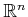. To define a distance function over any, however, certain axioms will have to be satisfied so that it coincides with our expectations based on Euclidean distance.

The following definition and axioms are used to create a function that converts a topological space into a metric space.5.1 A metric space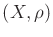is a topological spaceequipped with a function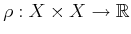such that for any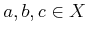:

1. Nonnegativity: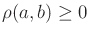.
2. Reflexivity: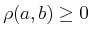if and only if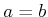.
3. Symmetry: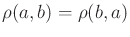.
4. Triangle inequality: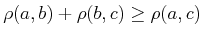.
The function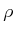defines distances between points in the metric space, and each of the four conditions onagrees with our intuitions about distance. The final condition implies thatis optimal in the sense that the distance from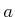to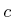will always be less than or equal to the total distance obtained by traveling through an intermediate point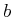on the way fromto.

Subsections
Steven M LaValle 2020-08-14